## Graphs-

• A graph is a collection of vertices connected to each other through a set of edges.
• The study of graphs is known as Graph Theory.

### Formal Definition

Formally,

A graph is defined as an ordered pair of a set of vertices and a set of edges.

G = (V, E)

Here, V is the set of vertices and E is the set of edges connecting the vertices.

### Example-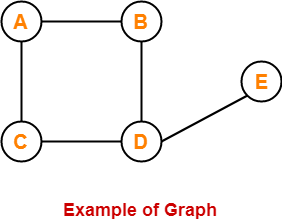In this graph,

V = { A , B , C , D , E }

E = { AB , AC , BD , CD , DE }

## Types of Graphs-

Various important types of graphs in graph theory are-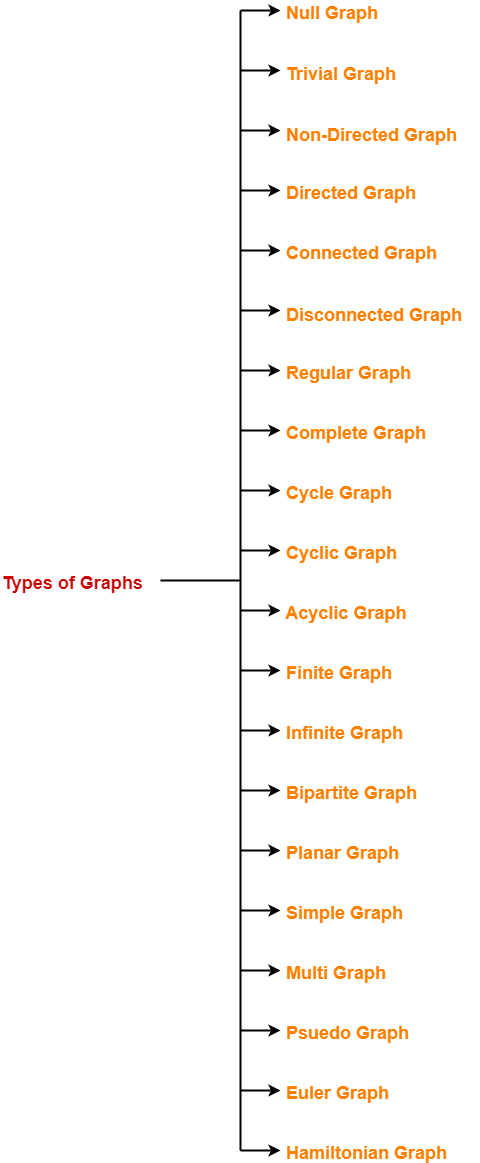1. Null Graph
2. Trivial Graph
3. Non-directed Graph
4. Directed Graph
5. Connected Graph
6. Disconnected Graph
7. Regular Graph
8. Complete Graph
9. Cycle Graph
10. Cyclic Graph
11. Acyclic Graph
12. Finite Graph
13. Infinite Graph
14. Bipartite Graph
15. Planar Graph
16. Simple Graph
17. Multi Graph
18. Pseudo Graph
19. Euler Graph
20. Hamiltonian Graph

## 1. Null Graph-

• A graph whose edge set is empty is called as a null graph.
• In  other words, a null graph does not contain any edges in it.

### Example-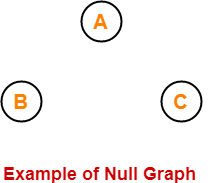Here,

• This graph consists only of the vertices and there are no edges in it.
• Since the edge set is empty, therefore it is a null graph.

## 2. Trivial Graph-

• A graph having only one vertex in it is called as a trivial graph.
• It is the smallest possible graph.

### Example-Here,

• This graph consists of only one vertex and there are no edges in it.
• Since only one vertex is present, therefore it is a trivial graph.

## 3. Non-Directed Graph-

• A graph in which all the edges are undirected is called as a non-directed graph.
• In other words, edges of an undirected graph do not contain any direction.

### Example-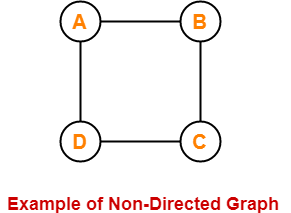Here,

• This graph consists of four vertices and four undirected edges.
• Since all the edges are undirected, therefore it is a non-directed graph.

## 4. Directed Graph-

• A graph in which all the edges are directed is called as a directed graph.
• In other words, all the edges of a directed graph contain some direction.
• Directed graphs are also called as digraphs.

### Example-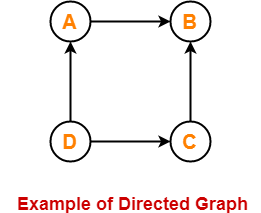Here,

• This graph consists of four vertices and four directed edges.
• Since all the edges are directed, therefore it is a directed graph.

## 5. Connected Graph-

• A graph in which we can visit from any one vertex to any other vertex is called as a connected graph.
• In connected graph, at least one path exists between every pair of vertices.

### Example-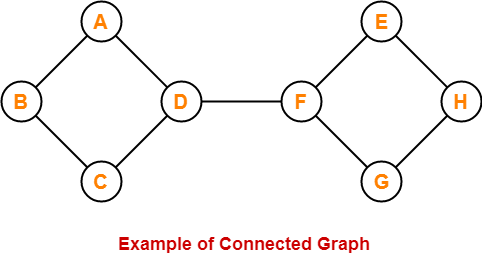Here,

• In this graph, we can visit from any one vertex to any other vertex.
• There exists at least one path between every pair of vertices.
• Therefore, it is a connected graph.

## 6. Disconnected Graph-

• A graph in which there does not exist any path between at least one pair of vertices is called as a disconnected graph.

### Example-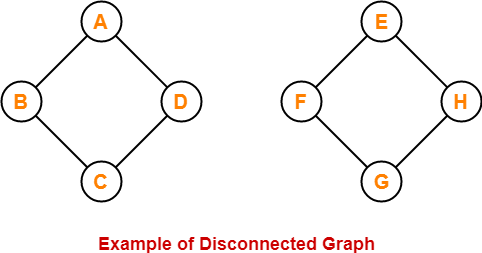Here,

• This graph consists of two independent components which are disconnected.
• It is not possible to visit from the vertices of one component to the vertices of other component.
• Therefore, it is a disconnected graph.

## 7. Regular Graph-

• A graph in which degree of all the vertices is same is called as a regular graph.
• If all the vertices in a graph are of degree ‘k’, then it is called as a “k-regular graph“.

### Examples-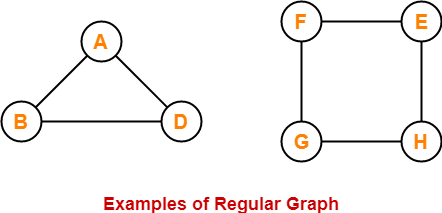In these graphs,

• All the vertices have degree-2.
• Therefore, they are 2-Regular graphs.

## 8. Complete Graph-

• A graph in which exactly one edge is present between every pair of vertices is called as a complete graph.
• A complete graph of ‘n’ vertices contains exactly nC2 edges.
• A complete graph of ‘n’ vertices is represented as Kn.

### Examples-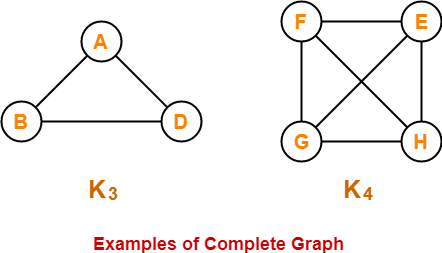In these graphs,

• Each vertex is connected with all the remaining vertices through exactly one edge.
• Therefore, they are complete graphs.

## 9. Cycle Graph-

• A simple graph of ‘n’ vertices (n>=3) and n edges forming a cycle of length ‘n’ is called as a cycle graph.
• In a cycle graph, all the vertices are of degree 2.

### Examples-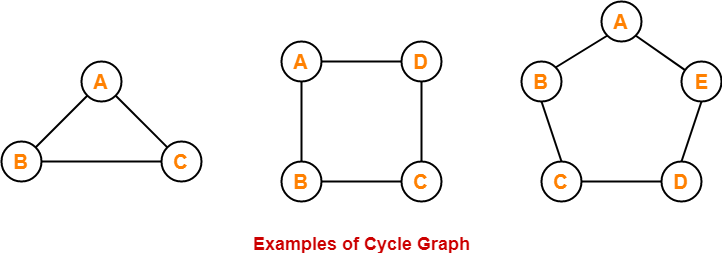In these graphs,

• Each vertex is having degree 2.
• Therefore, they are cycle graphs.

## 10. Cyclic Graph-

• A graph containing at least one cycle in it is called as a cyclic graph.

### Example-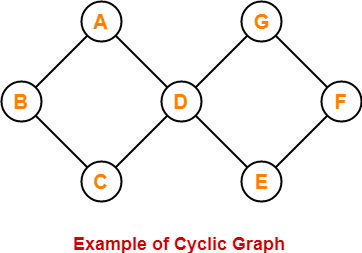Here,

• This graph contains two cycles in it.
• Therefore, it is a cyclic graph.

## 11. Acyclic Graph-

• A graph not containing any cycle in it is called as an acyclic graph.

### Example-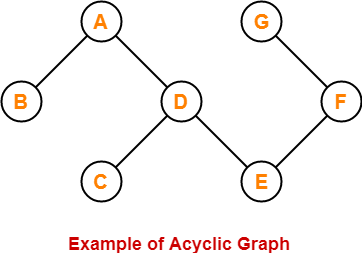Here,

• This graph do not contain any cycle in it.
• Therefore, it is an acyclic graph.

## 12. Finite Graph-

• A graph consisting of finite number of vertices and edges is called as a finite graph.

### Example-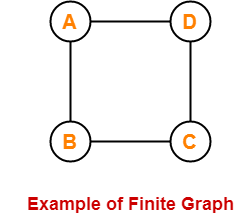Here,

• This graph consists of finite number of vertices and edges.
• Therefore, it is a finite graph.

## 13. Infinite Graph-

• A graph consisting of infinite number of vertices and edges is called as an infinite graph.

### Example-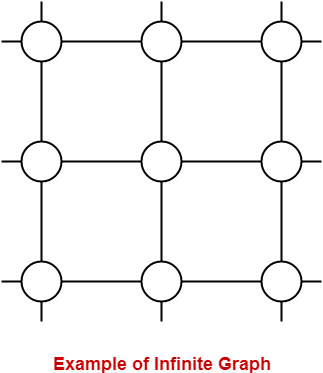Here,

• This graph consists of infinite number of vertices and edges.
• Therefore, it is an infinite graph.

## 14. Bipartite Graph-

A bipartite graph is a graph where-

• Vertices can be divided into two sets X and Y.
• The vertices of set X only join with the vertices of set Y.
• None of the vertices belonging to the same set join each other.

### Example-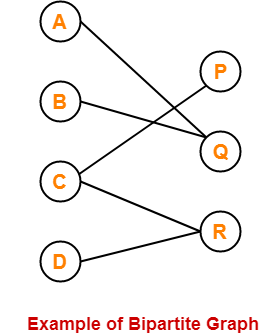## 15. Planar Graph-

• A planar graph is a graph that we can draw in a plane such that no two edges of it cross each other.

### Example-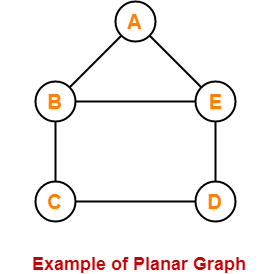Here,

• This graph can be drawn in a plane without crossing any edges.
• Therefore, it is a planar graph.

## 16. Simple Graph-

• A graph having no self loops and no parallel edges in it is called as a simple graph.

### Example-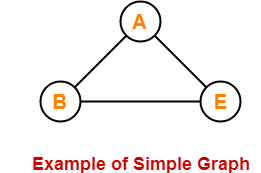Here,

• This graph consists of three vertices and three edges.
• There are neither self loops nor parallel edges.
• Therefore, it is a simple graph.

## 17. Multi Graph-

• A graph having no self loops but having parallel edge(s) in it is called as a multi graph.

### Example-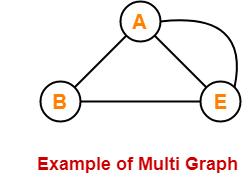Here,

• This graph consists of three vertices and four edges out of which one edge is a parallel edge.
• There are no self loops but a parallel edge is present.
• Therefore, it is a multi graph.

## 18. Pseudo Graph-

• A graph having no parallel edges but having self loop(s) in it is called as a pseudo graph.

### Example-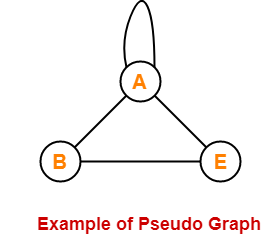Here,

• This graph consists of three vertices and four edges out of which one edge is a self loop.
• There are no parallel edges but a self loop is present.
• Therefore, it is a pseudo graph.

## 19. Euler Graph-

• Euler Graph is a connected graph in which all the vertices are even degree.

### Example-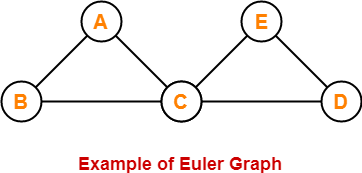Here,

• This graph is a connected graph.
• The degree of all the vertices is even.
• Therefore, it is an Euler graph.

## 20. Hamiltonian Graph-

• If there exists a closed walk in the connected graph that visits every vertex of the graph exactly once (except starting vertex) without repeating the edges, then such a graph is called as a Hamiltonian graph.

### Example-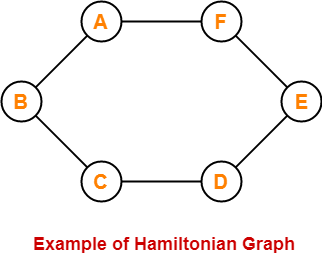Here,

• This graph contains a closed walk ABCDEFG that visits all the vertices (except starting vertex) exactly once.
• All the vertices are visited without repeating the edges.
• Therefore, it is a Hamiltonian Graph.

## Important Points-

• Edge set of a graph can be empty but vertex set of a graph can not be empty.
• Every polygon is a 2-Regular Graph.
• Every complete graph of ‘n’ vertices is a (n-1)-regular graph.
• Every regular graph need not be a complete graph.

## Remember-

The following table is useful to remember different types of graphs-

 Self-Loop(s) Parallel Edge(s) Graph Yes Yes Simple Graph No No Multi Graph No Yes Pseudo Graph Yes No

## Applications of Graph Theory-

Graph theory has its applications in diverse fields of engineering-

### 1. Electrical Engineering-

• The concepts of graph theory are used extensively in designing circuit connections.
• The types or organization of connections are named as topologies.
• Some examples for topologies are star, bridge, series and parallel topologies.

### 2. Computer Science-

Graph theory is used for the study of algorithms such as-

### 3. Computer Network-

The relationships among interconnected computers in the network follows the principles of graph theory.

### 4. Science-

Following structures are represented by graphs-

• Molecular structure of a substance
• Chemical structure of a substance
• DNA structure of an organism etc

### 5. Linguistics-

The parsing tree of a language and grammar of a language uses graphs.

### 6. Other Applications-

• Routes between the cities are represented using graphs.
• Hierarchical ordered information such as family tree are represented using special types of graphs called trees.

Next Article- Planar Graph

Get more notes and other study material of Graph Theory.

Watch video lectures by visiting our YouTube channel LearnVidFun.

## Types of Graphs-

Before you go through this article, make sure that you have gone through the previous article on various Types of Graphs in Graph Theory.

We have discussed-

• A graph is a collection of vertices connected to each other through a set of edges.
• The study of graphs is known as Graph Theory.## Bipartite Graph-

A bipartite graph is a special kind of graph with the following properties-

• It consists of two sets of vertices X and Y.
• The vertices of set X join only with the vertices of set Y.
• The vertices within the same set do not join.

### Bipartite Graph Example-

The following graph is an example of a bipartite graph-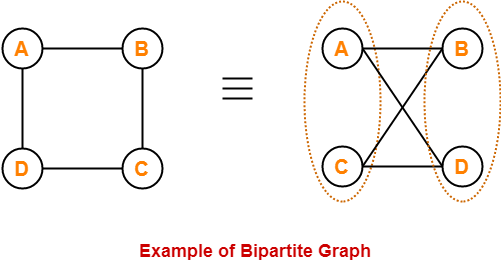Here,

• The vertices of the graph can be decomposed into two sets.
• The two sets are X = {A, C} and Y = {B, D}.
• The vertices of set X join only with the vertices of set Y and vice-versa.
• The vertices within the same set do not join.
• Therefore, it is a bipartite graph.

## Complete Bipartite Graph-

A complete bipartite graph may be defined as follows-

 A bipartite graph where every vertex of set X is joined to every vertex of set Y is called as complete bipartite graph. OR Complete bipartite graph is a bipartite graph which is complete. OR Complete bipartite graph is a graph which is bipartite as well as complete.

### Complete Bipartite Graph Example-

The following graph is an example of a complete bipartite graph-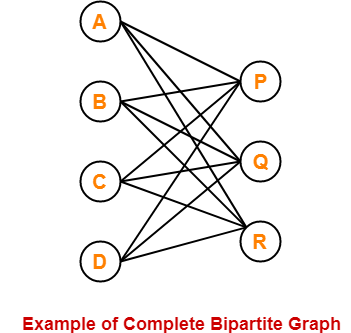Here,

• This graph is a bipartite graph as well as a complete graph.
• Therefore, it is a complete bipartite graph.
• This graph is called as K4,3.

## Bipartite Graph Chromatic Number-

To properly color any bipartite graph,

• Minimum 2 colors are required.
• This ensures that the end vertices of every edge are colored with different colors.
• Thus, bipartite graphs are 2-colorable.

### Note

If graph is bipartite with no edges, then it is 1-colorable.

### Example-

The chromatic number of the following bipartite graph is 2-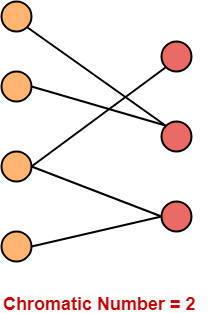## Bipartite Graph Properties-

Few important properties of bipartite graph are-

• Bipartite graphs are 2-colorable.
• Bipartite graphs contain no odd cycles.
• Every sub graph of a bipartite graph is itself bipartite.
• There does not exist a perfect matching for a bipartite graph with bipartition X and Y if |X| ≠ |Y|.
• In any bipartite graph with bipartition X and Y,

Sum of degree of vertices of set X = Sum of degree of vertices of set Y

## Bipartite Graph Perfect Matching-

 Number of complete matchings for Kn,n = n!

Given a bipartite graph G with bipartition X and Y,

• There does not exist a perfect matching for G if |X| ≠ |Y|.
• A perfect matching exists on a bipartite graph G with bipartition X and Y if and only if for all the subsets of X, the number of elements in the subset is less than or equal to the number of elements in the neighborhood of the subset.

### Maximum Number Of Edges-

• Any bipartite graph consisting of ‘n’ vertices can have at most (1/4) x n2 edges.
• Maximum possible number of edges in a bipartite graph on ‘n’ vertices = (1/4) x n2.

 Explanation Suppose the bipartition of the graph is (V1, V2) where |V1| = k and |V2| = n-k. The number of edges between V1 and V2 can be at most k(n-k) which is maximized at k = n/2. Thus, maximum 1/4 n2 edges can be present. Also, for any graph G with n vertices and more than 1/4 n2 edges, G will contain a triangle. This is not possible in a bipartite graph since bipartite graphs contain no odd cycles.

Also Read- Euler Graph & Hamiltonian Graph

## Problem-01:

Is the following graph a bipartite graph?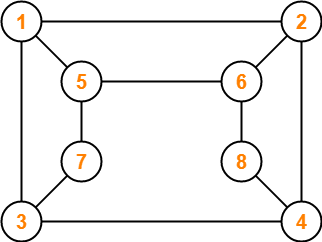## Solution-

The given graph may be redrawn as-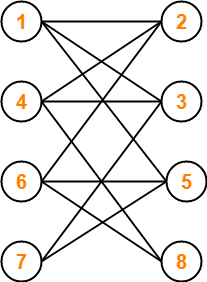Here,

• This graph consists of two sets of vertices.
• The two sets are X = {1, 4, 6, 7} and Y = {2, 3, 5, 8}.
• The vertices of set X are joined only with the vertices of set Y and vice-versa.
• Also, any two vertices within the same set are not joined.
• This satisfies the definition of a bipartite graph.

Therefore, Given graph is a bipartite graph.

## Problem-02:

The maximum number of edges in a bipartite graph on 12 vertices is _________?

## Solution-

We know, Maximum possible number of edges in a bipartite graph on ‘n’ vertices = (1/4) x n2.

Substituting n = 12, we get-

Maximum number of edges in a bipartite graph on 12 vertices

= (1/4) x (12)2

= (1/4) x 12 x 12

= 36

Therefore, Maximum number of edges in a bipartite graph on 12 vertices = 36.

To gain better understanding about Bipartite Graphs in Graph Theory,

Watch this Video Lecture

Next Article- Konigsberg Bridge Problem

Get more notes and other study material of Graph Theory.

Watch video lectures by visiting our YouTube channel LearnVidFun.

## Types of Graphs-

Before you go through this article, make sure that you have gone through the previous article on various Types of Graphs in Graph Theory.

We have discussed-

• A graph is a collection of vertices connected to each other through a set of edges.
• The study of graphs is known as Graph Theory.## Hamiltonian Graph-

A Hamiltonian graph may be defined as-

 If there exists a closed walk in the connected graph that visits every vertex of the graph exactly once (except starting vertex) without repeating the edges, then such a graph is called as a Hamiltonian graph. OR Any connected graph that contains a Hamiltonian circuit is called as a Hamiltonian Graph.

### Hamiltonian Graph Example-

The following graph is an example of a Hamiltonian graph-Here,

• This graph contains a closed walk ABCDEFA.
• It visits every vertex of the graph exactly once except starting vertex.
• The edges are not repeated during the walk.
• Therefore, it is a Hamiltonian graph.

Alternatively, there exists a Hamiltonian circuit ABCDEFA in the above graph, therefore it is a Hamiltonian graph.

## Hamiltonian Path-

• If there exists a walk in the connected graph that visits every vertex of the graph exactly once without repeating the edges, then such a walk is called as a Hamiltonian path.

OR

• If there exists a Path in the connected graph that contains all the vertices of the graph, then such a path is called as a Hamiltonian path.

### NOTE

In Hamiltonian path, all the edges may or may not be covered but edges must not repeat.

### Hamiltonian Path Examples-

Examples of Hamiltonian path are as follows-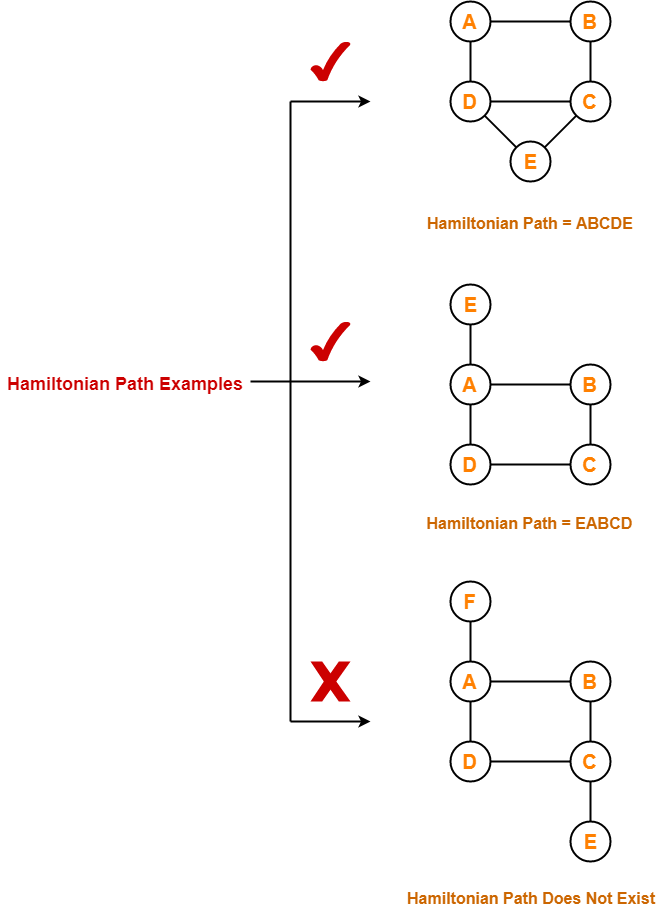## Hamiltonian Circuit-

Hamiltonian circuit is also known as Hamiltonian Cycle.

• If there exists a walk in the connected graph that visits every vertex of the graph exactly once (except starting vertex) without repeating the edges and returns to the starting vertex, then such a walk is called as a Hamiltonian circuit.

OR

• If there exists a Cycle in the connected graph that contains all the vertices of the graph, then that cycle is called as a Hamiltonian circuit.

OR

• A Hamiltonian path which starts and ends at the same vertex is called as a Hamiltonian circuit.

OR

• A closed Hamiltonian path is called as a Hamiltonian circuit.

### Hamiltonian Circuit Examples-

Examples of Hamiltonian circuit are as follows-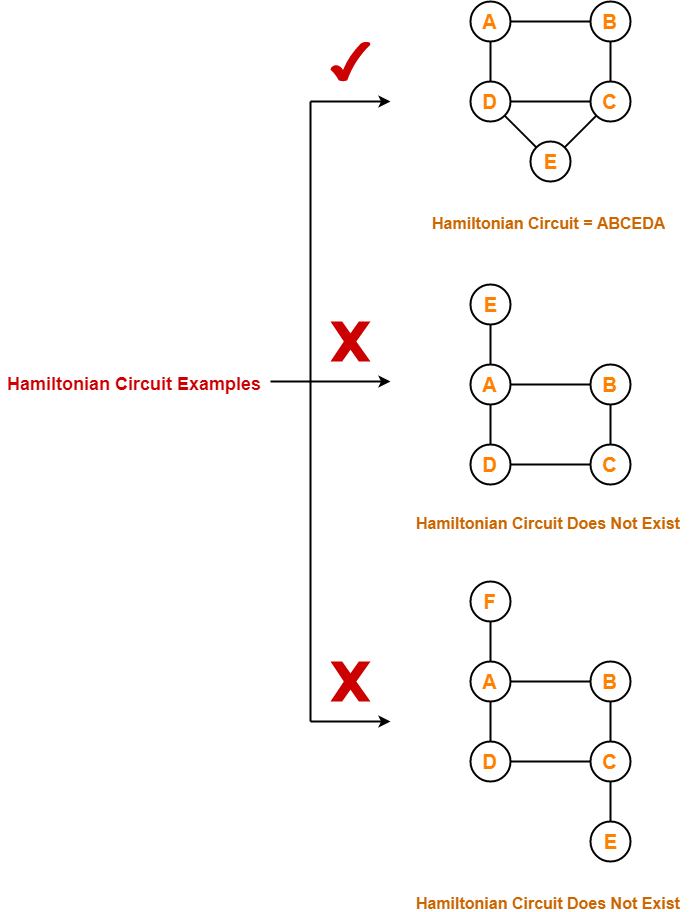## Important Notes-

• Any Hamiltonian circuit can be converted to a Hamiltonian path by removing one of its edges.
• Every graph that contains a Hamiltonian circuit also contains a Hamiltonian path but vice versa is not true.
• There may exist more than one Hamiltonian paths and Hamiltonian circuits in a graph.

## Problems-

Which of the following is / are Hamiltonian graphs?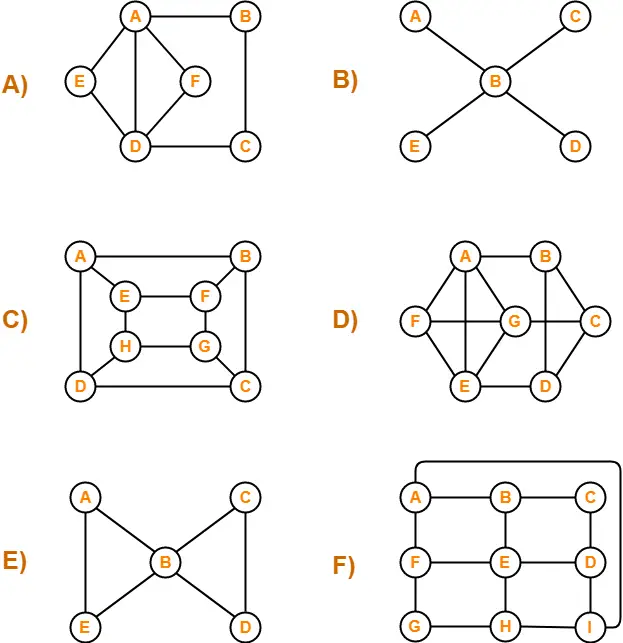## Solutions-

### A)

The graph neither contains a Hamiltonian path nor it contains a Hamiltonian circuit.

Since graph does not contain a Hamiltonian circuit, therefore It is not a Hamiltonian Graph.

### B)

The graph neither contains a Hamiltonian path nor it contains a Hamiltonian circuit.

Since graph does not contain a Hamiltonian circuit, therefore It is not a Hamiltonian Graph.

### C)

The graph contains both a Hamiltonian path (ABCDHGFE) and a Hamiltonian circuit (ABCDHGFEA).

Since graph contains a Hamiltonian circuit, therefore It is a Hamiltonian Graph.

### D)

The graph contains both a Hamiltonian path (ABCDEFG) and a Hamiltonian circuit (ABCDEFGA).

Since graph contains a Hamiltonian circuit, therefore It is a Hamiltonian Graph.

### E)

The graph neither contains a Hamiltonian path nor it contains a Hamiltonian circuit.

Since graph does not contain a Hamiltonian circuit, therefore It is not a Hamiltonian Graph.

### F)

The graph contains both a Hamiltonian path (ABCDEFGHI) and a Hamiltonian circuit (ABCDEFGHIA)

Since graph contains a Hamiltonian circuit, therefore It is a Hamiltonian Graph.

To gain better understanding about Hamiltonian Graphs in Graph Theory,

Watch this Video Lecture

Next Article- Bipartite Graph

Get more notes and other study material of Graph Theory.

Watch video lectures by visiting our YouTube channel LearnVidFun.

## Handshaking Theorem-

Handshaking Theorem is also known as Handshaking Lemma or Sum of Degree Theorem.

 In Graph Theory, Handshaking Theorem states in any given graph, Sum of degree of all the vertices is twice the number of edges contained in it.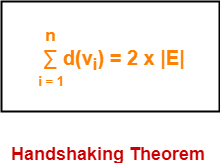The following conclusions may be drawn from the Handshaking Theorem.

In any graph,

• The sum of degree of all the vertices is always even.
• The sum of degree of all the vertices with odd degree is always even.
• The number of vertices with odd degree are always even.

## Problem-01:

A simple graph G has 24 edges and degree of each vertex is 4. Find the number of vertices.

## Solution-

Given-

• Number of edges = 24
• Degree of each vertex = 4

Let number of vertices in the graph = n.

Using Handshaking Theorem, we have-

Sum of degree of all vertices = 2 x Number of edges

Substituting the values, we get-

n x 4 = 2 x 24

n = 2 x 6

∴ n = 12

Thus, Number of vertices in the graph = 12.

## Problem-02:

A graph contains 21 edges, 3 vertices of degree 4 and all other vertices of degree 2. Find total number of vertices.

## Solution-

Given-

• Number of edges = 21
• Number of degree 4 vertices = 3
• All other vertices are of degree 2

Let number of vertices in the graph = n.

Using Handshaking Theorem, we have-

Sum of degree of all vertices = 2 x Number of edges

Substituting the values, we get-

3 x 4 + (n-3) x 2 = 2 x 21

12 + 2n – 6 = 42

2n = 42 – 6

2n = 36

∴ n = 18

Thus, Total number of vertices in the graph = 18.

## Problem-03:

A simple graph contains 35 edges, four vertices of degree 5, five vertices of degree 4 and four vertices of degree 3. Find the number of vertices with degree 2.

## Solution-

Given-

• Number of edges = 35
• Number of degree 5 vertices = 4
• Number of degree 4 vertices = 5
• Number of degree 3 vertices = 4

Let number of degree 2 vertices in the graph = n.

Using Handshaking Theorem, we have-

Sum of degree of all vertices = 2 x Number of edges

Substituting the values, we get-

4 x 5 + 5 x 4 + 4 x 3 + n x 2 = 2 x 35

20 + 20 + 12 + 2n = 70

52 + 2n = 70

2n = 70 – 52

2n = 18

∴ n = 9

Thus, Number of degree 2 vertices in the graph = 9.

## Problem-04:

A graph has 24 edges and degree of each vertex is k, then which of the following is possible number of vertices?

1. 20
2. 15
3. 10
4. 8

## Solution-

Given-

• Number of edges = 24
• Degree of each vertex = k

Let number of vertices in the graph = n.

Using Handshaking Theorem, we have-

Sum of degree of all vertices = 2 x Number of edges

Substituting the values, we get-

n x k = 2 x 24

k = 48 / n

Now,

• It is obvious that the degree of any vertex must be a whole number.
• So in the above equation, only those values of ‘n’ are permissible which gives the whole value of ‘k’.

Now, let us check all the options one by one-

• For n = 20, k = 2.4 which is not allowed.
• For n = 15, k = 3.2 which is not allowed.
• For n = 10, k = 4.8 which is not allowed.
• For n = 8, k = 6 which is allowed.

Thus, Option (D) is correct.

To gain better understanding about Handshaking Theorem,

Watch this Video Lecture

Next Article- Graph Isomorphism

Get more notes and other study material of Graph Theory.

Watch video lectures by visiting our YouTube channel LearnVidFun.

## Konigsberg-

• Konigsberg is the former name of a German city that is now in Russia.
• The following picture shows the inner city of Konigsberg with the river Pregel.
• The river Pregel divides the city into four land areas A, B, C and D.
• In order to travel from one part of the city to another, there exists seven bridges.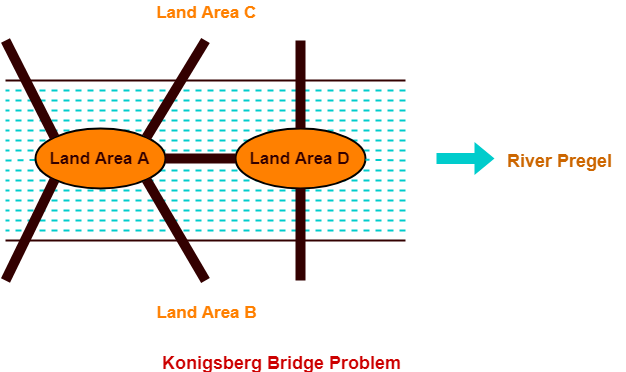## Konigsberg Bridge Problem-

Konigsberg Bridge Problem may be stated as-

 “Starting from any of the four land areas A, B, C, D, is it possible to cross each of the seven bridges exactly once and come back to the starting point without swimming across the river?”

## Konigsberg Bridge Problem Solution-

In 1735,

• A Swiss Mathematician Leon hard Euler solved this problem.
• He provided a solution to the problem and finally concluded that such a walk is not possible.

Euler represented the given situation using a graph as shown below-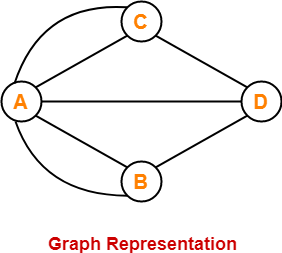In this graph,

• Vertices represent the landmasses.
• Edges represent the bridges.

Euler observed that when a vertex is visited during the process of tracing a graph,

• There must be one edge that enters into the vertex.
• There must be another edge that leaves the vertex.
• Therefore, order of the vertex must be an even number.

Based on this observation, Euler discovered that it depends on the number of odd vertices present in the network whether any network is traversable or not.

Euler found that only those networks are traversable that have either-

• No odd vertices (then any vertex may be the beginning and the same vertex will also be the ending point)
• Or exactly two odd vertices (then one odd vertex will be the starting point and other odd vertex will be the ending point)

Now,

• Since the Konigsberg network has four odd vertices, therefore the network is not traversable.
• Thus, It was finally concluded that the desired walking tour of Konigsberg is not possible.

### NOTE

If the citizens of Konigsberg decides to build an eighth bridge from A to C, then-

• It would be possible to walk without traversing any bridge twice.
• This is because then there will be exactly two odd vertices.

However, adding a ninth bridge will again make the walking tour once again impossible.

To gain better understanding about Konigsberg Bridge Problem,

Watch this Video Lecture

Next Article- Handshaking Theorem

Get more notes and other study material of Graph Theory.

Watch video lectures by visiting our YouTube channel LearnVidFun.